Gallery: Axis styles and grids

1) The default plot style is to show all four axes (closed)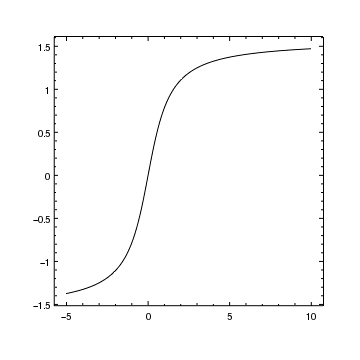Version: Postscript; PDF
```add_curve("atan.fits[cols X,Y]",["symbol.style","none"])
```

2) The open plot style only displays two axes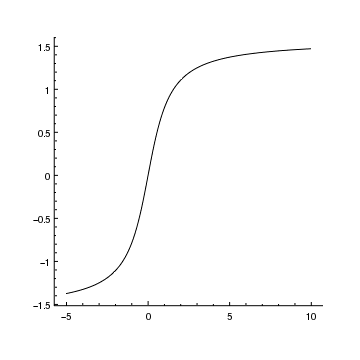Version: Postscript; PDF
```plt = ChipsPlot()
plt.style = "open"
crv = ChipsCurve()
crv.symbol.style = "none"
```

An alternative way of saying this in one command is:

```add_curve("atan.fits[cols X,Y]", ["plot.style", "open", "symbol.style", "none"])
```

3) The boxed plot style draws a box around the plot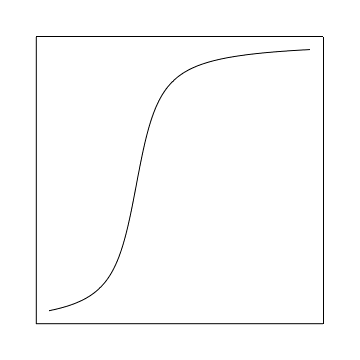Version: Postscript; PDF
```plt = ChipsPlot()
plt.style = "boxed"
crv = ChipsCurve()
crv.symbol.style = "none"

# We hide the axes to show the box surrounding the plot
hide_axis()

set_plot(["leftmargin",0.1,"bottommargin",0.1])
```

Although the axes have been hidden, the data is still displayed and the data range can still be changed; after the limits call below the plot changes to display the new range.

```chips> get_plot_xrange()
[-5.75, 10.75]
chips> get_plot_yrange()
[-1.5156271890074535, 1.6133540963661723]
chips> limits(Y_AXIS, -2, 2)
chips> get_plot_yrange()
[-2.0, 2.0]
```

4) Numeric labels (ticklabels) on all four axes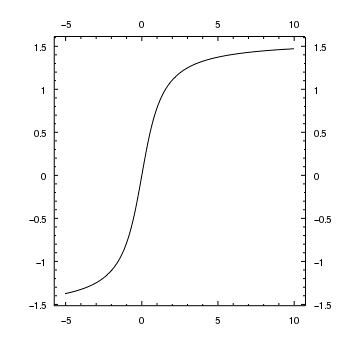Version: Postscript; PDF
```add_curve("atan.fits[cols X,Y]",["symbol.style","none"])

set_plot(["rightmargin",0.15])

set_axis("all",["ticklabel.visible",True,"ticklabel.offset",10])
```

The plot created by the add_curve call contains two axes: the X axis (ax1) at the bottom of the plot and the Y axis (ay1) at the left of the plot. There are also four borders which run along the edges of the plot: it is these that are controlled by the plot.style attribute, although you generally do not see the bx1 and by1 borders since they are hidden by the axes.

These borders are set up to automatically mirror the main axes; they are bound to ax1 or ay1 so that any changes to the limits, scaling, or tickmarks in the axes are also applied to the borders. This can be seen if you use the info_bound_axes call, which returns:

```chips> info_bound_axes()

Window [win1]
Frame [frm1]
plot1:bx1   ->   plot1:bx2
plot1:by1   ->   plot1:by2
plot1:ax1   ->   plot1:bx2
plot1:ay1   ->   plot1:by2

```

This says that the top and bottom borders (bx1 and bx2) are bound to each other and then to the X axis (ax1), and that the left and right borders (by1 and by2) are similarly bound to the Y axis (ay1).

The properties of borders can be changed using the set_axis call, either explicitly by giving a name - e.g.

```set_xaxis("bx2", ["ticklabel.color", "green"])
```

or implicitly by the use of the "all" value, as done in this example.

Unlike other ChIPS objects, borders can not be created nor can their names be changed. They are included in the output of info as shown below:

```chips> info()

Window [win1]
Frame [frm1]
Plot [plot1]   (0.15,0.15)  .. (0.85,0.90)
Border bottom [bx1]  top [bx2]  left [by1]  right [by2]
Curve [crv1]
X Axis [ax1]
Y Axis [ay1]

```

5) Rotating the numbers along an axis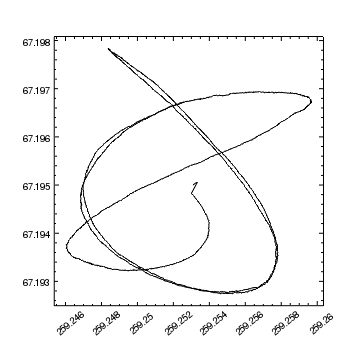Version: Postscript; PDF
```add_curve("aspect.fits[cols ra,dec]",["symbol.style","none"])

set_xaxis(["ticklabel.angle",40,"ticklabel.offset",16])
```

The properties of an axis can be found using one of the get_xaxis, get_yaxis, or get_axis routines. Here we display the X axis attributes after creating the plot above (due to the large number of attributes we suggest you use the ChIPS GUI to explore these settings; only when you are comfortable, or need to write a script, should you use the set_xaxis, set_xaxis, or set_xaxis commands):

```chips> get_xaxis()

automax = True
automin = True
color = default
depth = 100
id = None
label.color = default
label.font = helvetica
label.fontstyle = normal
label.halign = 0.5
label.size = 14
label.valign = 0.0
label.visible = True
majorgrid.color = default
majorgrid.style = 6
majorgrid.thickness = 1.0
majorgrid.visible = False
majortick.color = default
majortick.count = 6
majortick.interval = 10.0
majortick.length = 4
majortick.mode = limits
majortick.style = inside
majortick.thickness = 1.0
majortick.visible = True
minorgrid.color = default
minorgrid.style = 2
minorgrid.thickness = 1.0
minorgrid.visible = False
minortick.color = default
minortick.count = 4
minortick.interval = 5.0
minortick.length = 2
minortick.mode = nice
minortick.style = inside
minortick.thickness = 1.0
minortick.visible = True
offset.parallel = 0.0
offset.perpendicular = 40.0
thickness = 1.0
tickformat = %g
ticklabel.angle = 40.0
ticklabel.color = default
ticklabel.font = helvetica
ticklabel.fontstyle = normal
ticklabel.halign = -99.0
ticklabel.offset = 16
ticklabel.size = 12
ticklabel.style = outside
ticklabel.valign = -99.0
ticklabel.visible = True
tickstyle = inside
x.label.angle = 0.0
x.label.text =
x.stem = None
y.label.angle = None
y.label.text = None
y.stem = None

```

6) Changing the format used to display the labels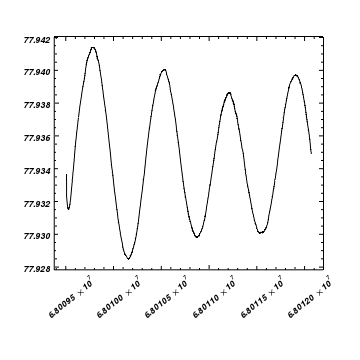Version: Postscript; PDF
```add_curve("aspect.fits[cols time,roll]",["symbol.style","none"])

set_plot(["bottommargin",0.25])

set_yaxis(["tickformat","%.3f"])

set_xaxis(["tickformat","%.5z","ticklabel.angle",40,"ticklabel.offset",30])

set_axis(["ticklabel.fontstyle","bolditalic"])
```

Axes can be labelled in a number of ways: there are a variety of formats that can be used - including sexagesimal notation - and the arrangement of major and minor tick marks can be adjusted, as shown in later examples (mode=interval and mode=count).

7) Using sexagesimal notation for the axis labels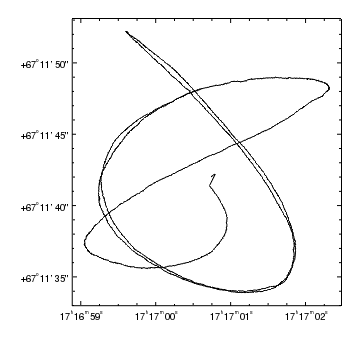Version: Postscript; PDF
```add_curve("aspect.fits[cols ra,dec]",["symbol.style","none"])

set_xaxis(["tickformat","ra"])
set_yaxis(["tickformat","dec"])
reposition_plot(0.2,0.15,0.95,0.95)
```

In this plot the X axis is drawn with Right Ascension increasing from left to right. The reverse_axes routine can be used to swap this axis: for example

```chips> reverse_axes(X_AXIS)
```

8) Changing the number of major tick marks (using mode=interval)

The major- and minor- tick marks on an axis have a mode parameter which determines how they are displayed (and can also change the display range). In this example we use the interval mode to specify the spacing of the major tick marks on both axes and the count mode to change the number of minor tick marks on the Y axis.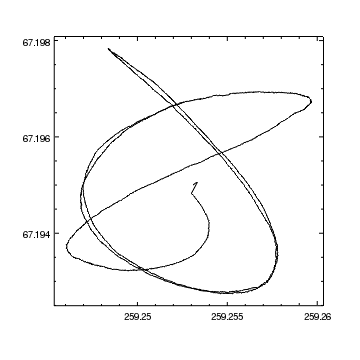Version: Postscript; PDF
```add_curve("aspect.fits[cols ra,dec]",["symbol.style","none"])

set_xaxis(["majortick.interval",0.005])
set_yaxis(["majortick.interval",0.002,"minortick.count",3])
```

We wish to set the X axis so that it has major tick marks - and hence numeric labels - every 0.005 degrees. To do this we use the interval value for majortick.mode and set the majortick.interval value to 0.005, which can be done with the following call

```set_xaxis(["majortick.mode", "interval", "majortick.interval", 0.005])
```

but is possible just with

```set_xaxis(["majortick.interval", 0.005])
```

since the set_xaxis and set_yaxis automatically fill in the correct majortick.mode value when the majortick.interval or majortick.count, attributes are set.

9) Changing the number of major tick marks (using mode=count)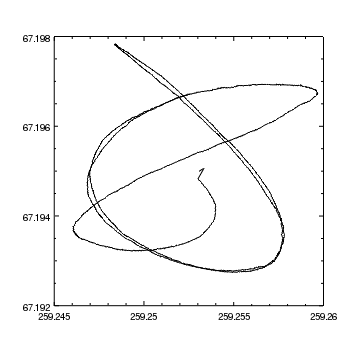Version: Postscript; PDF
```add_curve("aspect.fits[cols ra,dec]",["symbol.style","none"])

# Change both X and Y axes
set_axis(["majortick.count",4])

# Override the minortick.count setting for the Y axis
set_yaxis(["minortick.count",3])
```

As in the previous example, the set_axis call recognizes that setting the majortick.count value means that the majortick.mode should be set to count. At the end of the script the majortick and minortick fields of the Y axis look like:

```chips> get_yaxis().majortick

color = default
count = 4
interval = 10.0
length = 4
mode = count
style = inside
thickness = 1.0
visible = True

chips> get_yaxis().minortick

color = default
count = 3
interval = 5.0
length = 2
mode = nice
style = inside
thickness = 1.0
visible = True

```

10) Displaying a grid at the major tick mark locations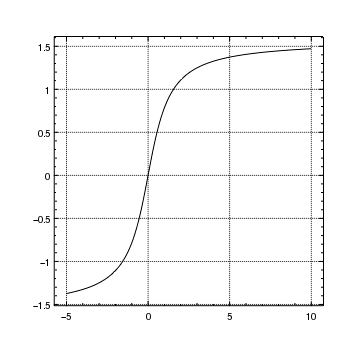Version: Postscript; PDF
```add_curve("atan.fits[cols X,Y]",["symbol.style","none"])

set_axis(["majorgrid.visible",True,"majorgrid.style","dot"])
```

11) Add a grid at the major and minor tick mark locations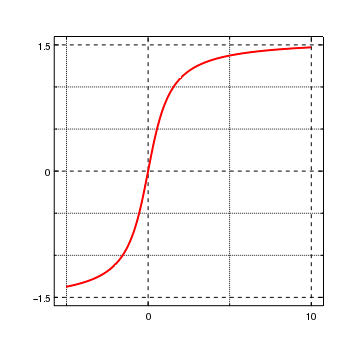Version: Postscript; PDF
```crv = ChipsCurve()
crv.symbol.style = "none"
crv.line.thickness = 2
crv.line.color = "red"

# Adjust the spacing of the major tick marks for each axis
set_xaxis(["majortick.interval",10,"minortick.count",1])
set_yaxis(["majortick.interval",1.5,"minortick.count",2])

# Set the visibility of the grids for both axes
set_axis(["majorgrid.visible",True,"minorgrid.visible",True])

limits(Y_AXIS,-1.6,1.6)
```

In this example we have created grid lines at the major and minor tick-mark locations. We use different line styles to improve visibility, and adjusted the spacing to the major and minor tick marks so as to change the grid spacing.

12) Grids work with logarithmically-scaled axes too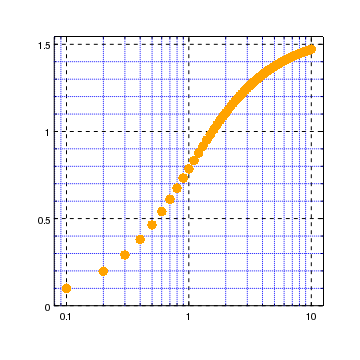Version: Postscript; PDF
```add_curve("atan.fits[cols x,y]")
crv = ChipsCurve()
crv.symbol.style = "circle"
crv.line.style = "noline"
crv.symbol.color = "orange"
set_curve(crv)

log_scale(X_AXIS)
limits(Y_AXIS,0,AUTO)

set_axis(["*.visible",True,"minorgrid.color","blue"])
set_yaxis(["majortick.interval",0.5])
```

In the previous example we used the command

```set_axis(["majorgrid.visible", True, "minorgrid.visible", True])
```

to display the axis grid lines. Here we use the short form *.visible to achieve the same effect (actually, this sets all components of the axis with a visible attribute, but by default these are already visible except for the majorgrid and minorgrid components).

13) Change the axis labels to use strings rather than numbers

In this example we plot temperature data for Massachusetts, taken from the Northeast Regional Climate Center, and stored in the file temperatures.txt:

```unix% cat temperatures.txt
# Month Temp LoTemp HiTemp
January 28.4 16.7 35.5
February 29.0 14.2 33.9
March 34.1 27.2 44.1
April 47.9 40.3 50.5
May 54.0 48.8 61.4
June 67.2 59.2 69.4
July 72.4 66.6 74.3
August 67.3 62.3 72.8
September 62.1 57.2 66.8
October 48.8 44.8 57.1
November 39.2 33.8 46.0
December 30.7 16.4 37.4
```

We use the set_arbitrary_tick_positions command to change the X axis labels from numbers to strings, and to display the Celcius temperature values on the right-hand Y axis.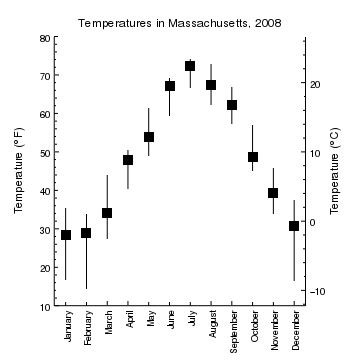Version: Postscript; PDF
```# Read in historical weather data for Massachusetts
xl = copy_colvals(tbl,"month")
y = copy_colvals(tbl,"temp")
yh = copy_colvals(tbl,"hitemp")
yl = copy_colvals(tbl,"lotemp")

# We need numeric X values for plotting the data
x = np.arange(len(y))

# Y errors
dyl = y - yl
dyh = yh - y

set_plot(["rightmargin",0.15,"style","open"])

# Change the X axis labels to days of the week
set_arbitrary_tick_positions("ax1",x,xl)

# Hide the minor tick marks on the X axis and rotate the ticklabels
ax = ChipsAxis()
ax.minortick.visible = False
ax.ticklabel.angle = 90
ax.ticklabel.halign = 1
ax.ticklabel.valign = 0.5
ax.ticklabel.offset = 5
set_xaxis(ax)

set_plot_ylabel(r"Temperature ({\textdegree}F)")
limits(Y_AXIS,10,80)

# Add a second Y axis to represent the temperature in degrees Celsius
# and make sure it covers the same data range as the original Y axis
limits(Y_AXIS,10,80)

yc = np.arange(-20,40,10)
yf = 9.0 * yc / 5 + 32

set_arbitrary_tick_positions("ay2",yf,yc)

set_plot_ylabel(r"Temperature ({\textdegree}C)")
set_plot_title("Temperatures in Massachusetts, 2008")
```

Since the data file contains the absolute temperature ranges for each month in the LoTemp and HiTemp columns, we use the read_file and copy_colvals routines to read in the data into arrays, and then calculate the required "error" arrays (we use the error bars of a curve to indicate the range of temperatures for each month).

Since we are going to add labels to the right-hand axis, we adjust the plot size and remove the top border by setting the style attribute to open in the set_plot call.

The first call to set_arbitrary_tick_positions changes the X axis (ax1), adding the labels in the xl array (i.e. the Month names read from the data file) at the positions given in the x array, which is the same array used when adding the curve. We rotate the new ticklabels, and adjust their alignment - using the set_xaxis call - so that they fit below the plot. Since the minor tick marks do not add anything for this axis we hide them by setting the ticklabel.visible attribute to 0.

In order to display the temperatures on both the Farenheit and Celsius scales, we add a second Y axis to the right-hand side of the plot. We first make sure that it displays exactly the same data range as the original Y axis; in this case 10 to 80. We then use set_arbitrary_tick_positions to place major tick marks at values of -20 to 30 degrees Centigrade, with a spacing of 10 degrees C. In this case, the minor tick marks are useful, so we do not hide them (unlike the X-axis case).

Since minor tick marks are only displayed between the labels created by set_arbitrary_tick_positions, we make sure that the yc array includes values outside the data range of the axis (namely 10 to 80). If the yc array had been created by saying

```yc = np.arange(-10, 30, 10)
```

instead then there would be no minor tick marks displayed above the 20 and below the -10 values on this axis.

Since there are now two Y axes, there are multiple coordinate systems, as shown in the output of info_coordinate:

```chips> info_coordinate()
Window [win1]
Frame [frm1]
Plot [plot1]
Coord Sys ID [ds0.0.0.3]
X Axis [ax1]
Y Axis [ay1]
Coord Sys ID [ds0.0.0.4]
X Axis [ax1]
Y Axis [ay2]

```

However, since they both cover the same numeric range, no matter what the labels on the right-hand axis say, we have

```chips> current_axis('ay1')
chips> get_plot_yrange()
[10.0, 80.0]
chips> current_axis('ay2')
chips> get_plot_yrange()
[10.0, 80.0]
```

14) Adding a background to a plot

Here we show how filled regions can be used to add a background color to a plot; this technique is also used in an axis-sharing example and when moving axes away from the plot.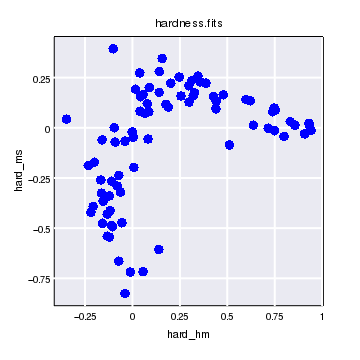Version: Postscript; PDF
```make_figure("hardness.fits",["line.style","noline"])
set_curve(["symbol.color","blue","symbol.style","circle"])

# Starting in CIAO 4.6, changing the fore/background preference
# values changes existing windows as well as new ones
set_preferences(["foreground.display","black","background.display","white"])

# Add a region, in plot-normalized coordinates, with a low depth value so
# that the grid lines will be drawn on top of it.
xr = [0, 1, 1, 0]
yr = [0, 0, 1, 1]

# Hide the tick marks, turn on the grid lines, then increase the
# grid spacing from 0.2 to 0.25.
set_axis("all",["majortick.visible",False,"minortick.visible",False])
axis = ChipsAxis()
axis.majorgrid.visible = True
axis.majorgrid.color = "white"
axis.majorgrid.thickness = 2
axis.majorgrid.style = "solid"
axis.majortick.interval = 0.25
set_axis(axis)
```

In this example the file - hardness.fits - only contains two columns, so these are used by the make_figure call to crearte a curve. The data is taken from the Chandra Source Catalog, using the obsid_search_csc script to download the basic source properties for ObsId 6436, and then the columns extracted using

```unix% punlearn obsid_search_csc
unix% obsid_search_csc 6436 6436.tsv
obsid_search_csc
obsid = 6436
outfile = 6436.tsv
columns = INDEF
root = ./
filetypes = regevt,pha,arf,rmf,lc,psf,regexp
catalog = csc1
verbose = 1
clobber = no
mode = ql

81 rows returned by query
81 Different Master Source(s).
1 Different Observation(s).

name                	ra          	dec         	obsid
CXO J032743.3+311723	 51.93047   	 31.28990   	 6436
...
CXO J032934.2+311743	 52.39284   	 31.29536   	 6436

If you use this data, please cite Evans et al 2010, ApJS 189, 37

unix% dmcopy "6436.tsv[opt kernel=text/tsv][cols hard_hm,hard_ms]" hardness.fits
```

The [opt kernel=text/tsv] specifier is required in the call to dmcopy to make sure that the Data Model reads in the tab-separated format used by the CSC correctly.

The foreground.display and background.display are set to match the scheme used for the hardcopy versions (the foreground.file and background.file preference settings), which can be useful when the opacity settings of objects are less than unity (in this example this is not the case).

To highlight the plot area, and so that the grid lines are visible, a region is added behind the plot since the default depth used for the plot and curve is 100. The coordinates used to create the plot are given in the plot-normalized coordinate system, which ensures that this region always covers the plot area, no matter if the plot limits are changed or the plot is resized - e.g. by calls like

```limits(XY_AXIS, -1, 1)
reposition_plot(0.2, 0.2, 0.95, 0.9)
```

Since the axes - and hence grid lines - are drawn on top of the region, the grid lines are visible even when set to white (the same color as the frame background). Note that when hiding the major and minor tick marks, the identifier was set to "all" in the set_axis call so that the borders (in this plot the axes at the top and right) as well as the axes (bottom and left), were changed.

The final setting in the set_axis - that of the majortick.interval attribute - is to change the spacing of the major tick marks, and hence the grid lines, to 0.25 by (for this data set the default spacing is 0.2). Earlier examples describe this in more detail: mode=interval and mode=count.